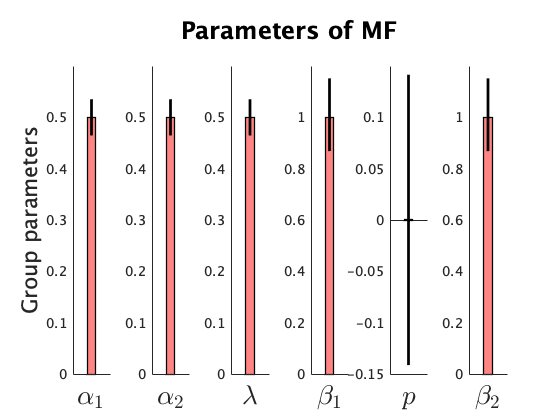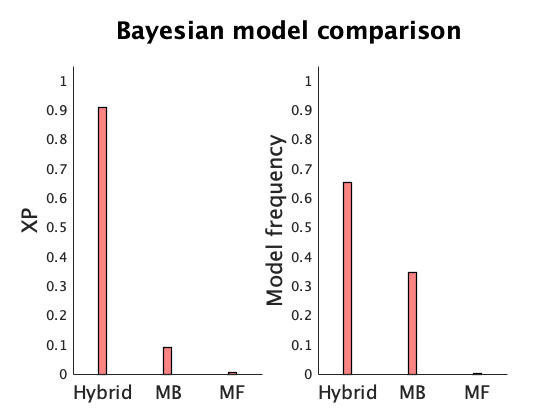# cbm manual¶

This document serves as the manual of cbm (computational and behavioral modeling) toolbox. cbm provides tools for hierarchical Bayesian inference (HBI). See the corresponding manuscript (here) for more information about the HBI and its comparison with other methods. You can download cbm here. In this manual, I present two well-known examples and explain how cbm tools should be used to perform Bayesian model comparison, parameter estimation and inference at the group level. To be able to follow this manual, you need to be familiar with matlab syntax (cbm will be published soon in other languages, particularly python).

# Why a new method?¶

Althoguh I don't aim to demonstrate benefits of the HBI over other methods here, I would like to give a hint that might motivate you to use the HBI. Bayesian methods are typically used for both parameter estimation and model comparison. Regarding the former, methods either perform heirarchical parameter estimation (i.e. building empirical priors based on group data) or non-hierarchical parameter estimation (i.e. fitting each subject independently). Of course, hierarchical parameter estimation is more accurate (and much less prone to subjective prior tuning). Regarding the model comparison, methods either take the model identity as a fixed effect (essentially making a very strong assumption that the same model is the true model for all subjects, so any difference between subjects is mereley due to noise) or as a random effect (different models might be true for different subjects). Of course, the random effects model comparison is better (particularly because of its robustness against outliers). However, prior to the HBI, no method was able to do both.

The HBI has important advantages for both parameter estimation and model comparison. The parameters estimated by the HBI show smaller errors compared to other methods. Model comparison by HBI is robust against outliers and is not biased towards overly simplistic models. Furthermore, the fully Bayesian approach of our theory enables researchers to make inference on group-level parameters by performing HBI t-test. See the corresponding paper theoretical and experimental results.

I assume that the current directory contains this manual and "codes" directory, as well as "example_RL_task" and "example_2step_task" directories. The codes directory contains all cbm matlab functions.

For using cbm tools, you need to know what is your model-space and code your own models. You can then use cbm to make inference. For this example, models code and data have been stored in the example_RL_task directory.

Suppose you have 40 subjects' choice data in a 2-armed bandit task, in which subjects chose between two actions and received binary outcomes.

All data have been stored in a mat-file called all_data.mat in a cell format, in which each cell contains choice data and outcomes for one subject. First, enter the example_RL_task directory and then load those data:

In :
% enter the example_RL_task folder,
% which contains data and models for this example
% (assuming that you are in the directory of the manual)

In :
% load data
data  = fdata.data;

% data for each subject
subj1 = data{1}; % subject 1
subj2 = data{2}; % subject 2
% and so on


For example, subj1 contains information of subject 1: subj1.actions are all actions and subj1.outcome are the corresponding outcomes.

Also suppose you have 2 candidate models. An RL model, which has a learning rate (alpha) and a decision noise (beta) parameter. The other model is a dual-alpha RL model with two separate learning rates for positive and negative prediction errors (alpha1 and alpha2, respectively) and a decision noise (beta) parameter. See matlab functions model_RL and model_dualRL as examples.

It is important to remember that cbm does not care how your model works! It assumes that your models take parameters and data (of one subject) as input and produce a log-probability of data given the parameters (i.e. log-likelihood function). Tools in cbm only require that the input and output of models follow a specific format:

loglik = model(parameters,subj)

For example, for the model_RL, we have:

loglik = model_RL(parameters,subj1)

here parameters is a vector, which its size depends on the number of parameters of the model_RL (for model_RL, its size is 2). subj1 is the structure containing data of subject 1 as indicated above. loglik is the log-likelihood of subj1 data given parameters, as computed by model_RL.

We now use cbm tools to fit models to data. For this example, we use the two models implemented in model_RL and model_dualRL together with the data stored in all_data.mat. Data stored in all_data is a synthetic dataset of 40 subjects. The data of the first 10 subjects are generated by model_RL and the data of the next 30 subjects are generated by model_dualRL. Note, however, that cbm is not meant to provide a collection of different computational models. You should code your models for questions of your interest yourself. The tools in cbm fit your models to your data and compare the models given the data.

In :
addpath(fullfile('..','codes'));

% load data from all_data.mat file
data  = fdata.data;


Before using cbm tools, it is always good to check whether the format of the models is compatible with the cbm. To do that, create a random vector parameters and call the models:

In :
% data of subject 1
subj1 = data{1};

parameters = randn(1,2);
F1 = model_RL(parameters,subj1)

parameters = randn(1,3);
F2 = model_dualRL(parameters,subj1)

F1 =

-200.9599

F2 =

-57.2434



Note that because parameters were randomly drawn, when you run the same code, it generates different values for F1 and F2. Also note that parameters are drawn from a normal distribution. In theory, RL models require constrained parameters (e.g. alpha between 0 and 1). In model_RL and model_dualRL, some transformations applied to the normally-distributed parameters to meet their theoretical bounds (see model_RL and model_dualRL). I'll explain later a bit more about transforming (normally-distributed) parameters in your models.

We checked the models with some random parameters. It is also good to check them with more extreme parameter values:

In :
parameters = [-10 10];
F1 = model_RL(parameters,subj1)

parameters = [-10 10 10];
F2 = model_dualRL(parameters,subj1)

F1 =

-654.4651

F2 =

-169.2246



Again, F1 and F2 should be real negative scalers.

After making sure that model_RL and model_dualRl work fine, we now use cbm tools to fit models to data.

First, we should run cbm_lap, which fits every model to each subject data separately (i.e. in a non-hierarchical fashion). cbm_lap employs Laplace approximation, which needs a normal prior for every parameter. We set zero as the prior mean. We also assume that the prior variance for all parameters is 6.25. This variance is large enough to cover a wide range of parameters with no excessive penalty (see supplementary materials of the reference article for more details on how this variance is calculated).

In :
v     = 6.25;
prior_RL = struct('mean',zeros(2,1),'variance',v); % note dimension of 'mean'
prior_dualRL = struct('mean',zeros(3,1),'variance',v); % note dimension of 'mean'


We also need to specify a file-address for saving the output of each model:

In :
fname_RL = 'lap_RL.mat';
fname_dualRL = 'lap_dualRL.mat';


Now we run cbm_lap for each model. Note that model_RL and model_dualRL are both in the current directory.

In :
cbm_lap(data, @model_RL, prior_RL, fname_RL);
% Running this command, prints a report on your matlab output
% (e.g. on the command window)

cbm_lap                                           18-Jun-2019 22:04:28
======================================================================
Number of samples: 40
Number of parameters: 2

Number of initializations: 14
----------------------------------------------------------------------
Subject: 01
Subject: 02
Subject: 03
Subject: 04
Subject: 05
Subject: 06
Subject: 07
Subject: 08
Subject: 09
Subject: 10
Subject: 11
Subject: 12
Subject: 13
Subject: 14
Subject: 15
Subject: 16
Subject: 17
Subject: 18
Subject: 19
Subject: 20
Subject: 21
Subject: 22
Subject: 23
Subject: 24
Subject: 25
Subject: 26
Subject: 27
Subject: 28
Subject: 29
Subject: 30
Subject: 31
Subject: 32
Subject: 33
Subject: 34
Subject: 35
Subject: 36
Subject: 37
Subject: 38
Subject: 39
Subject: 40
done :]


Also run cbm_lap for model_dualRL

In :
cbm_lap(data, @model_dualRL, prior_dualRL, fname_dualRL);
% Running this command, prints a report on your matlab output
% (e.g. on the command window)

cbm_lap                                           18-Jun-2019 22:04:35
======================================================================
Number of samples: 40
Number of parameters: 3

Number of initializations: 21
----------------------------------------------------------------------
Subject: 01
Subject: 02
Subject: 03
Subject: 04
Subject: 05
Subject: 06
Subject: 07
Subject: 08
Subject: 09
Subject: 10
Subject: 11
Subject: 12
Subject: 13
Subject: 14
Subject: 15
Subject: 16
Subject: 17
Subject: 18
Subject: 19
Subject: 20
Subject: 21
Subject: 22
Subject: 23
Subject: 24
Subject: 25
Subject: 26
Subject: 27
Subject: 28
Subject: 29
Subject: 30
Subject: 31
Subject: 32
Subject: 33
Subject: 34
Subject: 35
Subject: 36
Subject: 37
Subject: 38
Subject: 39
Subject: 40
done :]


Let's take a look at the file saved by the cbm_lap:

In :
fname = load('lap_RL.mat');
cbm   = fname.cbm;
% look at fitted parameters
cbm.output.parameters

ans =

-0.9099   -0.1596
-2.2959    0.2281
-1.8879    0.0641
-2.9416    0.6446
0.1626   -1.7082
-2.3983   -0.2423
-2.2385   -0.3139
-1.1035   -0.1045
-0.1508   -1.0967
-3.1319    0.3766
-1.6312    2.8806
-1.9657    1.4585
1.3684    1.3809
0.2462    1.2869
0.5822    1.6409
0.1195    0.7961
0.2869    1.3080
-0.9798    2.4429
-0.2855    1.3087
0.6944    2.0278
1.3887    1.4371
0.0819    1.0805
-0.5053    1.9617
0.4317    1.4263
0.1695    0.8347
0.6204   -0.8997
0.0504    2.2729
-0.2048   -0.2258
0.4743    1.2425
0.5359    0.7142
1.0363    0.0334
0.7106    0.2387
-1.4016    1.7652
0.0332    2.4478
-0.8369    1.4053
-0.0262    0.6052
0.1145    1.2742
0.3787    1.6922
0.0006    2.0756
1.6417    0.6821



Note that these values are normally-distributed parameters (I'll explain later how to obtain bounded parameters). The order of parameters depend on how they have been coded in the corresponding model. In model_RL, the first parameter is alpha and the second one is beta. Therefore, here the first column corresponds to alpha and the second one to beta.

Now we can do hierarchical Bayesian inference using cbm_hbi. cbm_hbi needs 4 inputs. The good news is that you already have all of them!

In :
% 1st input: data for all subjects
data  = fdata.data;

% 2nd input: a cell input containing function handle to models
models = {@model_RL, @model_dualRL};
% note that by handle, I mean @ before the name of the function

% 3rd input: another cell input containing file-address to files saved by cbm_lap
fcbm_maps = {'lap_RL.mat','lap_dualRL.mat'};
% note that they corresponds to models (so pay attention to the order)

% 4th input: a file address for saving the output
fname_hbi = 'hbi_RL_dualRL.mat';


Now, we are ready to run cbm_hbi:

In :
cbm_hbi(data,models,fcbm_maps,fname_hbi);
% Running this command, prints a report on your matlab output
% (e.g. on the command window)

cbm_hbi_hbi                                       18-Jun-2019 22:04:43
Running hierarchical bayesian inference (HBI)...

HBI has been initialized according to
lap_RL.mat [for model 1]
lap_dualRL.mat [for model 2]

Number of samples: 40
Number of models: 2
======================================================================
Iteration 01
Iteration 02
model frequencies (percent)
model 1: 50.5| model 2: 49.5|
dL:    6.62
dm:   49.48
dx:    0.22
Iteration 03
model frequencies (percent)
model 1: 48.6| model 2: 51.4|
dL:    2.14
dm:    1.91
dx:    0.12
Iteration 04
model frequencies (percent)
model 1: 46.3| model 2: 53.7|
dL:    3.09
dm:    2.30
dx:    0.13
Iteration 05
model frequencies (percent)
model 1: 43.3| model 2: 56.7|
dL:    4.28
dm:    3.04
dx:    0.16
Iteration 06
model frequencies (percent)
model 1: 39.4| model 2: 60.6|
dL:    4.91
dm:    3.84
dx:    0.18
Iteration 07
model frequencies (percent)
model 1: 35.6| model 2: 64.4|
dL:    4.10
dm:    3.81
dx:    0.17
Iteration 08
model frequencies (percent)
model 1: 32.7| model 2: 67.3|
dL:    3.42
dm:    2.93
dx:    0.15
Iteration 09
model frequencies (percent)
model 1: 31.2| model 2: 68.8|
dL:    2.08
dm:    1.47
dx:    0.11
Iteration 10
model frequencies (percent)
model 1: 30.5| model 2: 69.5|
dL:    1.40
dm:    0.72
dx:    0.12
Iteration 11
model frequencies (percent)
model 1: 30.0| model 2: 70.0|
dL:    0.99
dm:    0.53
dx:    0.10
Iteration 12
model frequencies (percent)
model 1: 29.6| model 2: 70.4|
dL:    0.65
dm:    0.34
dx:    0.08
Iteration 13
model frequencies (percent)
model 1: 29.4| model 2: 70.6|
dL:    0.44
dm:    0.21
dx:    0.06
Iteration 14
model frequencies (percent)
model 1: 29.3| model 2: 70.7|
dL:    0.30
dm:    0.13
dx:    0.04
Iteration 15
model frequencies (percent)
model 1: 29.2| model 2: 70.8|
dL:    0.21
dm:    0.07
dx:    0.03
Iteration 16
model frequencies (percent)
model 1: 29.2| model 2: 70.8|
dL:    0.15
dm:    0.04
dx:    0.02
Iteration 17
model frequencies (percent)
model 1: 29.1| model 2: 70.9|
dL:    0.11
dm:    0.02
dx:    0.01
Iteration 18
model frequencies (percent)
model 1: 29.1| model 2: 70.9|
dL:    0.08
dm:    0.01
dx:    0.01
Iteration 19
model frequencies (percent)
model 1: 29.1| model 2: 70.9|
dL:    0.06
dm:    0.00
dx:    0.01
Converged :]


Runnig cbm_hbi writes a report on your standard output (often the screen). On every iteration, cbm_hbi reports model frequency, which is the estimate of how many individual datasets (i.e. subjects) is explained by each model (in percent). Furthermore, on every iteration, there are 3 metrics showing the changes relative to the previous iteration: dL is the change in the log-likelihood of all data given the model space (more specifically a variational approximation of log-likelihood); dm is the (percentage of) change in model frequencies; dx indicates changes in (normalized value of) parameters. Although either of these measures or their combination can be used as stopping criteria, cbm_hbi uses dx as the stopping criteria. By default, cbm_hbi stops when dx<0.01.

Let's now take a look at the saved file:

In :
fname_hbi = load('hbi_RL_dualRL.mat');
cbm   = fname_hbi.cbm;
cbm.output

ans =

struct with fields:

parameters: {2x1 cell}
responsibility: [40x2 double]
group_mean: {[-1.8854 -0.0098]  [1.0678 -0.2944 1.1510]}
group_hierarchical_errorbar: {[0.2954 0.0409]  [0.1183 0.1038 0.0912]}
model_frequency: [0.2913 0.7087]
exceedance_prob: [0.0041 0.9959]
protected_exceedance_prob: [NaN NaN]



Almost all useful parameters are stored in cbm.output, which we explain them here.

First, let's look at model_frequency, which is the HBI estimate of how much each model is expressed across the group:

In :
model_frequency = cbm.output.model_frequency

model_frequency =

0.2913    0.7087



Note that the model_frequency is normalized here (so it sums to 1 across all models). Also note that the order depends on the order of models fed into to HBI as input. Therefore, in this example, HBI estimated that about 29% of all subjects are explained by the model_RL and about 71% by the model_dualRL.

Now let's take a look at estimated group mean stored in cbm.output.group_mean.

In :
group_mean_RL = cbm.output.group_mean{1}
% group mean for parameters of model_RL

group_mean_dualRL = cbm.output.group_mean{2}
% group mean for parameters of model_dualRL

group_mean_RL =

-1.8854   -0.0098

group_mean_dualRL =

1.0678   -0.2944    1.1510



Note that these are normally distributed parameters. That's the reason that group learning rate (which is typically constrained to be between zero and one) is negative here. This is because HBI (and other tools in the cbm) assume that parameters are normally distributed. Therefore, if you have a model with some constraints on parameters (e.g. an RL), you should transform the normally distributed parameter in your model function. To make this point clear, take a look at the model_RL function. On the first two lines of this function, you see these codes:

nd_alpha = parameters(1); % normally-distributed alpha

alpha = 1/(1+exp(-nd_alpha)); % alpha (transformed to be between zero and one)

Here, nd_alpha is the normally-distributed parameter passed to the model (for example by cbm tools). Before using it, the model transformed it to alpha, which is bounded between 0 and 1 and is served as the effective learning rate. To do this, a sigmoid function has been used:

$\text{sigmoid}(x)=\frac{1}{1+e^{-x}}$

which is illustrated here:

In :
x = -5:.1:5;
y = 1./(1+exp(-x));
plot(x,y);
title('y = sigmoid(x)'); xlabel('x'); ylabel('y');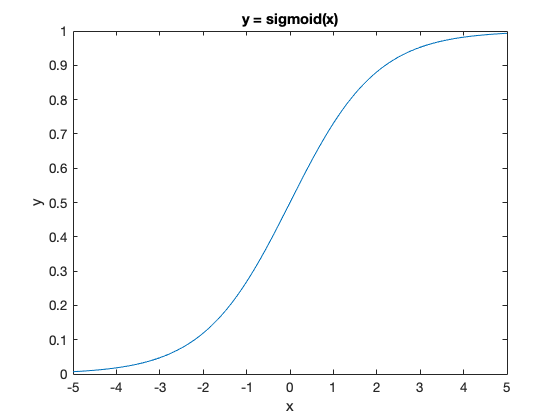As you see, sigmoid is a monotonically increasing function, which transforms its input (x) to an output (y) between 0 and 1. Therefore, if you want to obtain the parameters of your model in their theoretical range (e.g. a learning rate between 0 and 1), you should apply the same transformation (e.g. the sigmoid) to the normally distributed parameter (e.g. to the group_mean_RL(1)).

The second parameter of the model_RL is the decision noise parameter, which is theoretically constrained to be positive. For transforming this one, we did an exponential-transformation to the second parameter passed to the model_RL:

nd_beta = parameters(2);

beta = exp(nd_beta);

The HBI also quantifies (hierarchical) errorbar of the group_mean parameters, which is saved in cbm.output.group_hierarchical_errorbar:

In :
group_errorbar_RL = cbm.output.group_hierarchical_errorbar{1};
group_errorbar_dualRL = cbm.output.group_hierarchical_errorbar{2};


You can use the group_mean and group_hierarchical_errorbar values to plot group parameters, or use cbm_hbi_plot to plot the main outputs of the HBI.

In :
% 1st input is the file-address of the file saved by cbm_hbi
fname_hbi = 'hbi_RL_dualRL.mat';

% 2nd input: a cell input containing model names
model_names = {'RL', 'Dual RL'};
% note that they corresponds to models (so pay attention to the order)

% 3rd input: another cell input containing parameter names of the winning model
param_names = {'\alpha^+','\alpha^-','\beta'};
% note that '\alpha^+' is in the latex format, which generates a latin alpha

% 4th input: another cell input containing transformation function associated with each parameter of the winning model
transform = {'sigmoid','sigmoid','exp'};
% note that if you use a less usual transformation function, you should pass the handle here (instead of a string)

cbm_hbi_plot(fname_hbi, model_names, param_names, transform)
% this function creates a model comparison plot (exceednace probability and model frequency) as well as
% a plot of transformed parameters of the most frequent model.

plotting the group parameters of the most frequenct modelThere is no protected exceedance probability as cbm_hbi_null has not been executed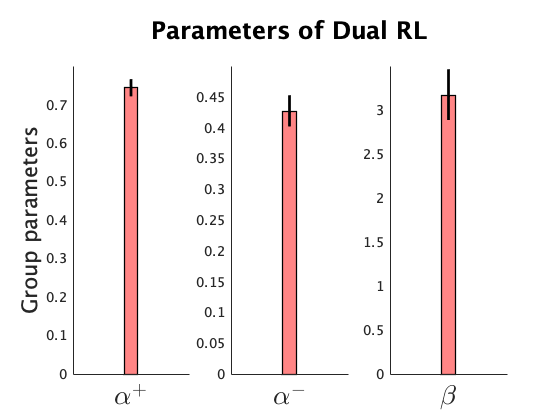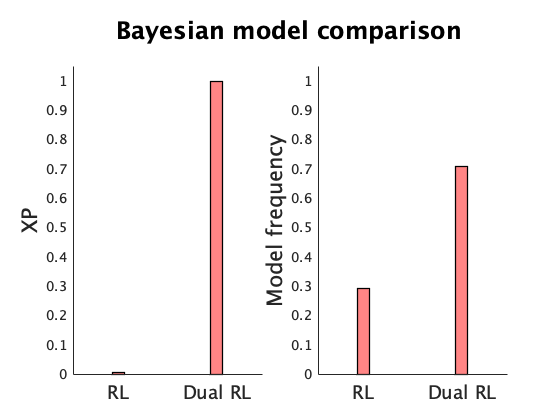Similar to a t-test, you can use the hierarchical errorbars to make an inference about a parameter at the population level. We explain that feature in the next example.

The value of individual parameters are saved in the cbm.output.parameters

In :
parameters_RL = cbm.output.parameters{1};
parameters_dualRL = cbm.output.parameters{2};


Also you can look at the estimated responsibility that each model generated each individual dataset. Across models, responsibilities sum to 1 for each subject.

In :
responsibility = cbm.output.responsibility

responsibility =

0.9755    0.0245
0.9992    0.0008
0.9993    0.0007
0.9996    0.0004
0.9990    0.0010
0.9995    0.0005
0.9923    0.0077
0.9934    0.0066
0.9972    0.0028
1.0000    0.0000
0.0000    1.0000
0.0000    1.0000
0.0000    1.0000
0.0000    1.0000
0.0000    1.0000
0.0002    0.9998
0.0000    1.0000
0.0000    1.0000
0.0000    1.0000
0.0000    1.0000
0.0000    1.0000
0.0000    1.0000
0.0000    1.0000
0.0000    1.0000
0.0007    0.9993
0.6553    0.3447
0.0000    1.0000
0.5644    0.4356
0.0000    1.0000
0.0007    0.9993
0.3221    0.6779
0.1548    0.8452
0.0000    1.0000
0.0000    1.0000
0.0000    1.0000
0.0002    0.9998
0.0000    1.0000
0.0000    1.0000
0.0000    1.0000
0.0012    0.9988



The first and second columns indicate the responsibility of model_RL and model_dualRL in generating the corresponding subject data, respectively.

Look at the estimated responsibility of model_dualRL:

In :
plot(responsibility(:,2)); ylim([-.1 1.1])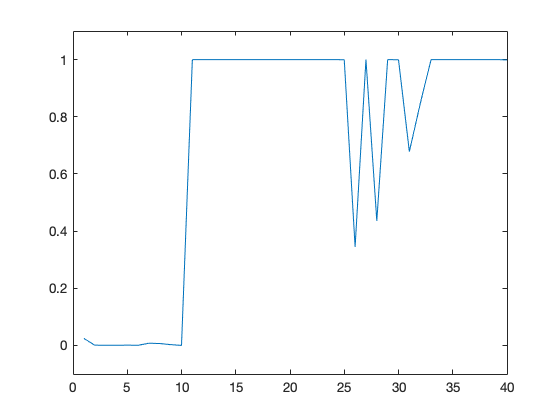As you see, the estimated responsibility of model_dualRL for the first 10 subjects is almost zero. These results make sense as this is a synthetic dataset and the first 10 subjects are actually generated using model_RL. The next 30 individual datasets are generated using model_dualRL.

Let's also take a look at exceedance probability, a metric typically used for model selection.

In :
xp = cbm.output.exceedance_prob

xp =

0.0041    0.9959



The exceedance probability indicates the probability that each model is the most likely model across the group.

A more useful metric is called protected exceedance probability, which also takes into account the null hypothesis that no model in the model space is most likely across the population (i.e. any difference between model frequencies is due to chance).

In :
pxp = cbm.output.protected_exceedance_prob

pxp =

NaN   NaN



As you see, this is currently only NaN values. This is because for computing protected exceedance probabilities, the HBI should be re-run under the (prior) null hypothesis.

This is how you can do it:

In :
fdata = load('all_data.mat');
data  = fdata.data;

fname_hbi = 'hbi_RL_dualRL';

% 1st input is data,
% 2nd input is the file-address of the file saved by cbm_hbi
cbm_hbi_null(data,fname_hbi);
% Running this command, prints a report on your matlab output
% (e.g. on the command window)

cbm_hbi_hbi                                       18-Jun-2019 22:05:01
Running hierarchical bayesian inference (HBI)- null mode...

HBI has been initialized according to
lap_RL.mat [for model 1]
lap_dualRL.mat [for model 2]

Number of samples: 40
Number of models: 2
======================================================================
Iteration 01
Iteration 02
dL:    5.07
dx:    0.15
Iteration 03
dL:    1.14
dx:    0.05
Iteration 04
dL:   -0.08
dx:    0.02
Iteration 05
dL:    0.06
dx:    0.01
Iteration 06
dL:    0.08
dx:    0.01
Converged :]


cbm_hbi_null saves a new file 'hbi_RL_dualRL_null.mat' and it also updates the cbm in hbi_RL_dualRL.mat.

Load again hbi_RL_dualRL.mat and look at the protected exceedance probability

In :
fname_hbi = load('hbi_RL_dualRL.mat');
cbm   = fname_hbi.cbm;
pxp   = cbm.output.protected_exceedance_prob

pxp =

0.0041    0.9959



Note that here values of xp and pxp are not really different (their difference is very small). In many datasets, however, their difference might be quite substantial.

# Example 2: Two-step Markov decision task¶

We now use cbm tools for computational modeling in the two-step Markov decision task introduced by Daw et al. (2011). This task is a well-known paradigm to distinguish two behavioral modes, model-based and model-free learning. Daw et al. have proposed three reinforcement learning accounts, a model-based, a model-free and their hybrid (which nests the other two and combines their estimates according to a weight parameter), to disentangle contribution of these two behavioral modes on choices.

For this example, we use an empirical dataset reported in Piray et al. (2016) (20 subjects for this example), which is stored in the example_2step_task directory.

The hybrid, model-based and model-free algorithms contain 7, 4 and 6 parameters, respectively. Please see Daw et al. for formal description of the models.

loglik = model(parameters,subj);

In :
% assuming that the current directory is example_RL_task

% load data from all_data.mat file
data  = fdata.data;

subj1 = data{1};
subj1

subj1 =

struct with fields:

choice1: [201x1 double]
transit: [201x1 double]
state2: [201x1 double]
choice2: [201x1 double]
outcome: [201x1 double]
description: {5x1 cell}



See subj1.description for a description of information stored for each subject.

Now, you should call cbm_lap with each model separately.

In :
prior_mb = struct('mean',zeros(4,1),'variance',6.25);
fname_mb = 'lap_mb.mat';
cbm_lap(data, @model_mb, prior_mb, fname_mb);
% Running this command, prints a report on your matlab output
% (e.g. on the command window)


If you have many subjects or a model with many parameters, the cbm_lap can take a long time to fit all of them. If you have access to cluster computing, however, you can run cbm_lap in parallel for subjects. For example, here we fit model_mf to the data of only subject 1:

In :
% create a directory for individual output files:
mkdir('lap_subjects');

% 1st input: data
% now the input data should be the data of subject 1
data_subj = data(1);

% 2nd input: function handle of model (i.e. @model_mf)

% 3rd input: a prior struct. The size of mean should
% be equal to the number of parameters
prior_mf = struct('mean',zeros(6,1),'variance',6.25);

% 4th input: output file
% note that here the output is associated with subject 1
% we save all output files in the lap_subjects directory
fname_mf_subj = fullfile('lap_subjects','lap_mf_1.mat');

cbm_lap(data_subj, @model_mf, prior_mf, fname_mf_subj);

Warning: Directory already exists.
cbm_lap                                           18-Jun-2019 22:05:04
======================================================================
Number of samples: 1
Number of parameters: 6

Number of initializations: 42
----------------------------------------------------------------------
Subject: 01
done :]


After all jobs finished, you should call cbm_lap_aggregate to aggregate individual files:

In :
% first make a list of lap_mf_* files:
fname_subjs = cell(20,1);
for n=1:length(fname_subjs)
fname_subjs{n} = fullfile('lap_subjects',['lap_mf_' num2str(n) '.mat']);
end
fname_subjs

fname_subjs =

20x1 cell array

{'lap_subjects/lap_mf_1.mat' }
{'lap_subjects/lap_mf_2.mat' }
{'lap_subjects/lap_mf_3.mat' }
{'lap_subjects/lap_mf_4.mat' }
{'lap_subjects/lap_mf_5.mat' }
{'lap_subjects/lap_mf_6.mat' }
{'lap_subjects/lap_mf_7.mat' }
{'lap_subjects/lap_mf_8.mat' }
{'lap_subjects/lap_mf_9.mat' }
{'lap_subjects/lap_mf_10.mat'}
{'lap_subjects/lap_mf_11.mat'}
{'lap_subjects/lap_mf_12.mat'}
{'lap_subjects/lap_mf_13.mat'}
{'lap_subjects/lap_mf_14.mat'}
{'lap_subjects/lap_mf_15.mat'}
{'lap_subjects/lap_mf_16.mat'}
{'lap_subjects/lap_mf_17.mat'}
{'lap_subjects/lap_mf_18.mat'}
{'lap_subjects/lap_mf_19.mat'}
{'lap_subjects/lap_mf_20.mat'}



Now specify the final output file-address and call cbm_lap_aggregate

In :
fname_mf = 'lap_mf.mat';
cbm_lap_aggregate(fname_subjs,fname_mf);
% Running this command prints a report on your matlab output
% (e.g. on the command window)

Aggregation is done over 20 subjects :]


You see that lap_mf.mat is saved by cbm_lap_aggregate. Similarly, you can fit model_hybrid to data using cbm_lap. I did that and saved lap_hybrid.mat.

Now that we have fitted models to data using cbm_lap, we can run cbm_hbi. Note that you can configure the algorithm using optional inputs:

In :
% 1st input: data for all subjects
data  = fdata.data;

% 2nd input: a cell input containing function handle to models
models = {@model_hybrid, @model_mb, @model_mf};
% note that by handle, I mean @ before the name of the function

% 3rd input: another cell input containing file-address to files saved by cbm_lap
fcbm_maps = {'lap_hybrid.mat','lap_mb.mat','lap_mf.mat'};
% note that they corresponds to models (so pay attention to the order)

% 4th input: a file address for saving the output
fname_hbi = 'hbi_2step.mat';

cbm_hbi(data,models,fcbm_maps,fname_hbi);
% Running this command prints a report on your matlab output
% (e.g. on the command window)


Now, we look at the hbi_2step.mat file saved by the cbm_hbi

In :
fname_hbi = load('hbi_2step.mat');
cbm   = fname_hbi.cbm;


First, take a look at model frequencies:

In :
cbm.output.model_frequency

ans =

0.6531    0.3469    0.0000



As you see the hybrid model takes about 65% of responsibility. Now let's take a look at the exceedance probability

In :
cbm.output.exceedance_prob

ans =

0.9102    0.0897    0.0000



Note that for computing the protected exceedance probability, the HBI should be re-run under the (prior) null hypothesis cbm_hbi_null.

Next, we look at the parameters of the hybrid model:

In :
cd(fullfile('..','example_2step_task'))

% 1st input is the file-address of the file saved by cbm_hbi
fname_hbi = 'hbi_2step.mat';

% 2nd input: a cell input containing model names
model_names = {'Hybrid', 'MB', 'MF'};
% note that they corresponds to models (so pay attention to the order)

% 3rd input: another cell input containing parameter names of the winning model
param_names = {'\alpha_1','\alpha_2','\lambda','\beta_1','w','p','\beta_2'};

% 4th input: another cell input containing transformation function associated with each parameter of the winning model
transform = {'sigmoid','sigmoid','sigmoid','exp','sigmoid','none','exp'};
% note that no transformation applied to parameter p (i.e perserveration) in the hybrid model, so we just pass 'none'.

cbm_hbi_plot(fname_hbi, model_names, param_names, transform)
% this function creates a model comparison plot (exceednace probability and model frequency) as well as
% a plot of transformed parameters of the most frequent model.

plotting the group parameters of the most frequenct modelThere is no protected exceedance probability as cbm_hbi_null has not been executed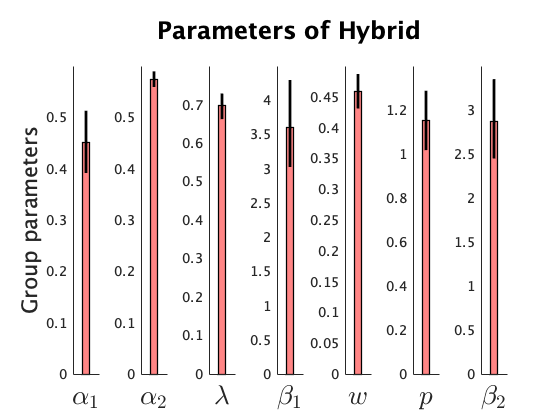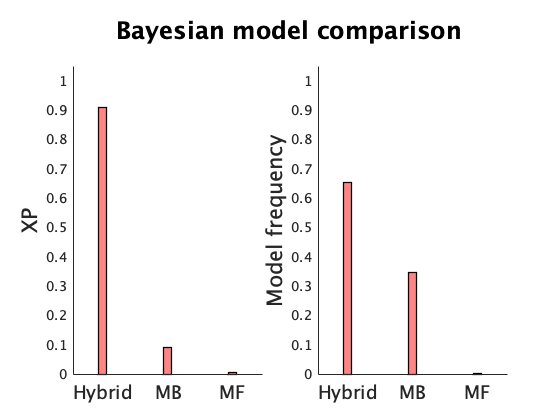A critical parameter of the hybrid model is the weight parameter, which indicates the degree to which choices influenced by model-based and model-free values. Since the weight parameter is also constrained to be between 0 (i.e. pure model-free) and 1 (i.e. pure model-based), the normally distributed parameter has been transformed in model_hybrid using the sigmoid function.

Similar to a t-test, you can use the hierarchical errorbars to make an inference about a parameter at the population level. For example, suppose you are interested to test whether the subjects show significantly more model-based than model-free behavior. In terms of the hybrid model, this can be examined by testing whether the (transformed) weight parameter is significantly different from 0.5 (which indicates equal contribution of model-based and model-free values). Since sigmoid function transforms 0 to 0.5, we should test whether the normally distributed weight parameter is significantly different from 0. cbm_hbi_ttest performs this inference according to a Student's t-distribution:

In :
% 1st input: the fitted cbm by cbm_hbi
fname_hbi = 'hbi_2step';

% 2nd input: the index of the model of interest in the cbm file
k = 1; % as the hybrid is the first model fed to cbm_hbi

% 3rd input: the test will be done compared with this value (i.e. this value indicates the null hypothesis)
m = 0; % here the weight parameter should be tested against m=0

% 4th input: the index of the parameter of interest
i = 5; % here the weight parameter is the 5th parameter of the hybrid model

[p,stats] = cbm_hbi_ttest(cbm,k,m,i)

p =

0.1667

stats =

struct with fields:

tstat: -1.4585
pval: 0.1667
df: 14.0626



We see that the p-value is not smaller than 0.05, so there is no signigicant evidence that the weight is different from 0. In other words, there is no significant evidence that subjects are more influenced by model-based or model-free values.

As another example, let's see whether the perseveration paramater is significantly different from 0 (parameter p in the above plot). This parameter indicates whether subjects repeat their choices (or avoid if p<0) regardless of the estimated values. This parameter has not been transformed, so the test should be against m=0.

In :
% 1st input: the fitted cbm by cbm_hbi
fname_hbi = 'hbi_2step';

% 2nd input: the index of the model of interest in the cbm file
k = 1; % as the hybrid is the first model fed to cbm_hbi

% 3rd input: the test will be done compared with this value (i.e. this value indicates the null hypothesis)
m = 0; % here the perseveration parameter should be tested against m=0

% 4th input: the index of the parameter of interest
d = 6; % here the perseveration parameter is the 6th parameter of the hybrid model

[p,stats] = cbm_hbi_ttest(cbm,k,m,d)

p =

6.1737e-07

stats =

struct with fields:

tstat: 8.5369
pval: 6.1737e-07
df: 14.0626



Therefore, the perseveration parameter is significantly larger than zero (p<0.001).

In this example, the model-free model does not take any responsibility. In this situation, the HBI assigns prior values to the individual parameters. This prior value is 0 before transformation. Therefore, for parameters bounded in the unit range (e.g. learning rate), this prior value (after transformation) is 0.5, for decision noise parameter is 1 and for the reservation parameter is 0.

In :
% 1st input is the file-address of the file saved by cbm_hbi
fname_hbi = 'hbi_2step.mat';

% 2nd input: a cell input containing model names
model_names = {'Hybrid', 'MB', 'MF'};
% note that they corresponds to models (so pay attention to the order)

% 3rd input: another cell input containing parameter names of the winning model
param_names = {'\alpha_1','\alpha_2','\lambda','\beta_1','p','\beta_2'};

% 4th input: another cell input containing transformation function associated with each parameter of the winning model
transform = {'sigmoid','sigmoid','sigmoid','exp','none','exp'};
% note that no transformation applied to parameter p (i.e perserveration) in the hybrid model, so we just pass 'none'.

cbm_hbi_plot(fname_hbi, model_names, param_names, transform, 3)
% this function creates a model comparison plot (exceednace probability and model frequency) as well as
% a plot of transformed parameters of the most frequent model.

There is no protected exceedance probability as cbm_hbi_null has not been executed